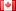# Revision history [back]

### complex conjugate of a variable

I attempted to construct the complex conjugate of a variable thus -
x = var('x') f = x*conjugate(x) f({x:2+2i}) this throws a SyntaxError, although the f({x:2+2i}) case works perfectly.2 No.2 RevisionDSM5242 ●21 ●68 ●112

### complex conjugate of a variable

I attempted to construct the complex conjugate of a variable thus -

x = var('x')
f = x*conjugate(x)
f({x:2+2i})


this throws a SyntaxError, although the f({x:2+2i}) case works perfectly.3 No.3 RevisionDSM5242 ●21 ●68 ●112

### complex conjugate of a variable

I attempted to construct the complex conjugate of a variable thus -

x = var('x')
f = x*conjugate(x)
f({x:2+2i})


this throws a SyntaxError, although the f({x:2+2i}) case works perfectly.

### complex conjugate of a variable

I attempted to construct the complex conjugate of a variable thus -

x = var('x')
f = x*conjugate(x)
f({x:2+2i})


this throws a SyntaxError, although the f({x:2+2i})f({x:2}) case works perfectly.